Next: Exercises Up: Multi-Dimensional Motion Previous: Projectile Motion with Air

# Charged Particle Motion in Electric and Magnetic Fields

Consider a particle of mass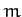and electric charge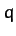moving in the uniform electric and magnetic fields,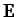and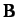. Suppose that the fields are crossed'' (i.e., perpendicular to one another), so that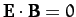.

The force acting on the particle is given by the familiar Lorentz law: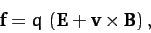(194)

whereis the particle's instantaneous velocity. Hence, from Newton's second law, the particle's equation of motion can be written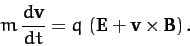(195)

It turns out that we can eliminate the electric field from the above equation by transforming to a different inertial frame. Thus, writing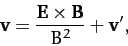(196)

Equation (195) reduces to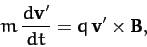(197)

where we have made use of a standard vector identity (see Section A.10), as well as the fact that. Hence, we conclude that the addition of an electric field perpendicular to a given magnetic field simply causes the particle to drift perpendicular to both the electric and magnetic field with the fixed velocity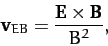(198)

irrespective of its charge or mass. It follows that the electric field has no effect on the particle's motion in a frame of reference which is co-moving with the so-called E-cross-B velocity given above.

Let us suppose that the magnetic field is directed along the-axis. As we have just seen, in the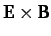frame, the particle's equation of motion reduces to Equation (197), which can be written: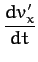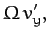(199)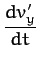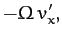(200)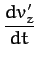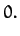(201)

Here,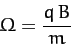(202)

is the so-called cyclotron frequency. Equations (199)-(201) can be integrated to give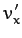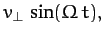(203)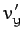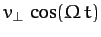(204)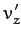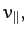(205)

where we have judiciously chosen the origin of time so as to eliminate any phase offset in the arguments of the above trigonometrical functions. According to Equations (203)-(205), in theframe, our charged particle gyrates at the cyclotron frequency in the plane perpendicular to the magnetic field with some fixed speed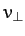, and drifts parallel to the magnetic field with some fixed speed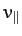. The fact that the cyclotron frequency is positive for positively charged particles, and negative for negatively charged particles, just means that oppositely charged particles gyrate in opposite directions in the plane perpendicular to the magnetic field.

Equations (203)-(205) can be integrated to give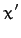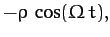(206)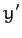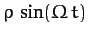(207)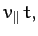(208)

where we have judiciously chosen the origin of our coordinate system so as to eliminate any constant offsets in the above equations. Here,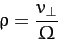(209)

is called the Larmor radius. Equations (206)-(208) are the equations of a spiral of radius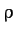, aligned along the direction of the magnetic field (i.e., the-direction).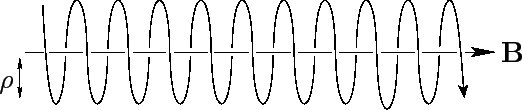We conclude that the general motion of a charged particle in crossed electric and magnetic field is a combination ofdrift [see Equation (198)] and spiral motion aligned along the direction of the magnetic field--see Figure 12. Particles drift parallel to the magnetic field with constant speeds, and gyrate at the cyclotron frequency in the plane perpendicular to the magnetic field with constant speeds. Oppositely charged particles gyrate in opposite directions.Next: Exercises Up: Multi-Dimensional Motion Previous: Projectile Motion with Air
Richard Fitzpatrick 2011-03-31